Next: 5.6 Computer Calculation of Up: 5. Area Previous: 5.4 Logarithms.   Index

# 5.5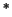Brouncker's Formula For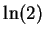The following calculation ofis due to William Brouncker (1620-1684)[22, page 54].

Let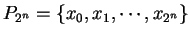denote the regular partition of the interval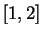into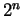equal subintervals. Let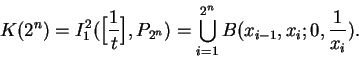We can construct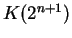from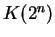by adjoining a box of width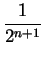to the top of each box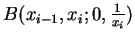that occurs in the definition of(see figures a) and b)).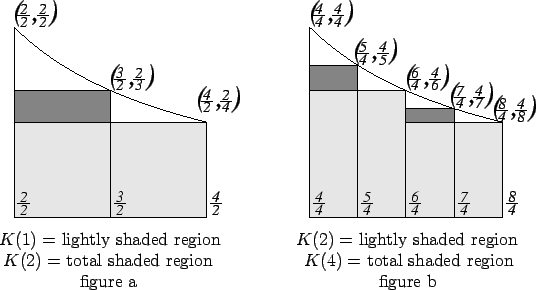We have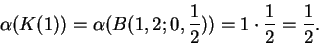From figure a) we see that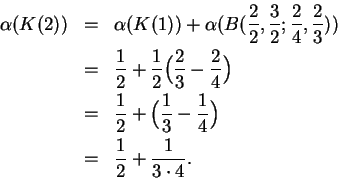From figure b) we see that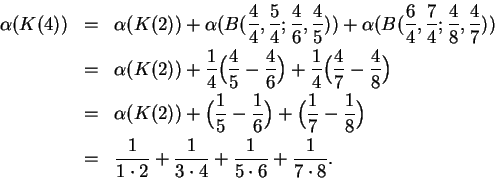In general we will find that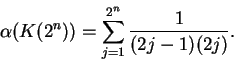Now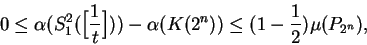i.e.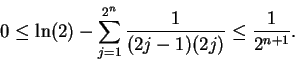Thus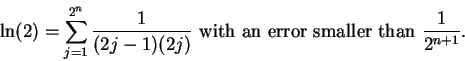We can think ofas being given by the infinite sum"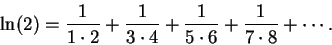(5.82)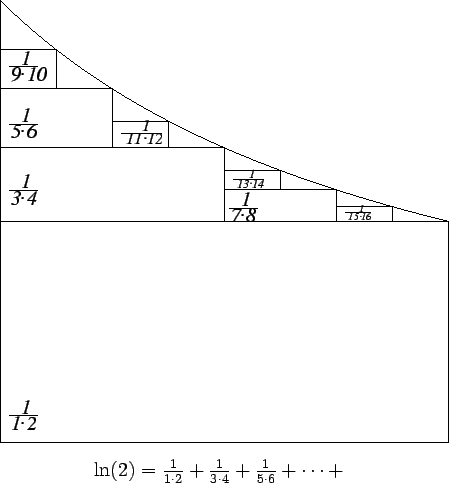Equation (5.82) is sometimes called Mercator's expansion for, after Nicolaus Mercator, who found the result sometime near 1667 by an entirely different method.

Brouncker's calculation was published in 1668, but was done about ten years earlier [22, pages 56-56].

Brouncker's formula above is an elegant result, but it is not very useful for calculating: it takes too many terms in the sum to get much accuracy. Today, when a logarithm can be found by pressing a button on a calculator, we tend to think of" as being a known number, and of Brouncker's formula as giving a closed form" for the sum of the infinite series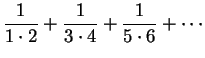.Next: 5.6 Computer Calculation of Up: 5. Area Previous: 5.4 Logarithms.   Index
Ray Mayer 2007-09-07